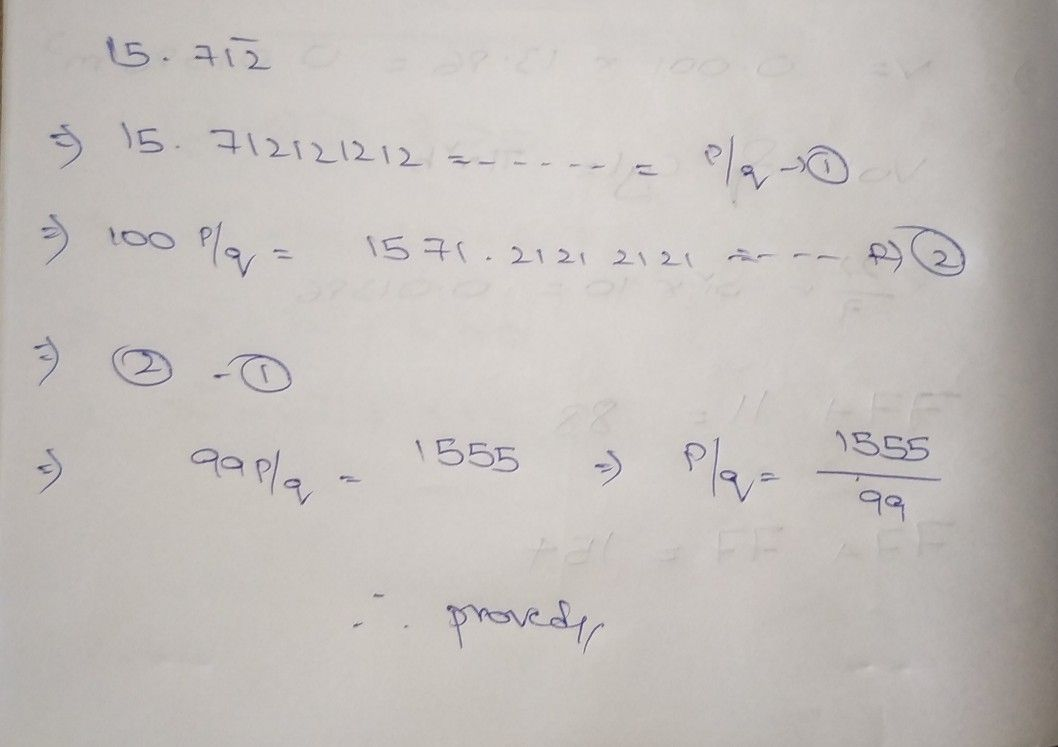Symbol
Problem$7$ $60$ poress $15.7\bar{12} 5$ the foom $\square$ where p and $9y$ $2$ $0+09^{8}$ tegers and $9≠○$ $1$ $1$ $=$
7th-9th grade
Other
SolutionQanda teacher - kamesh